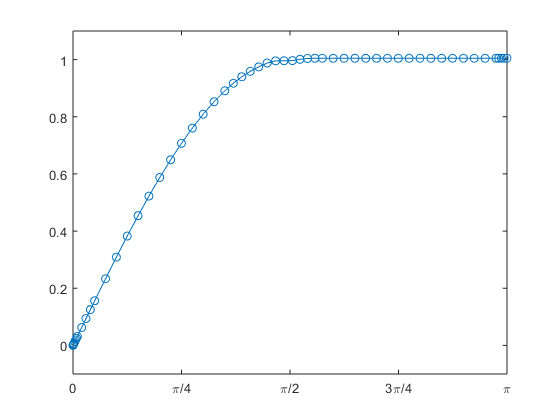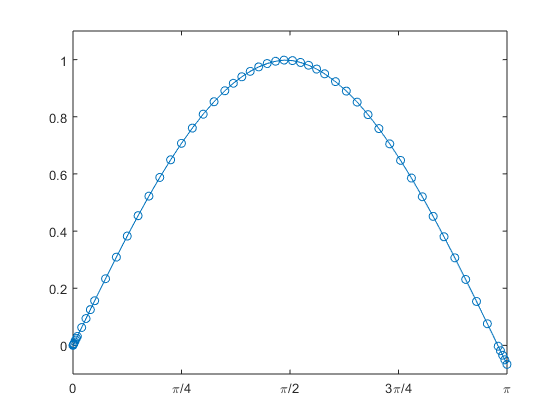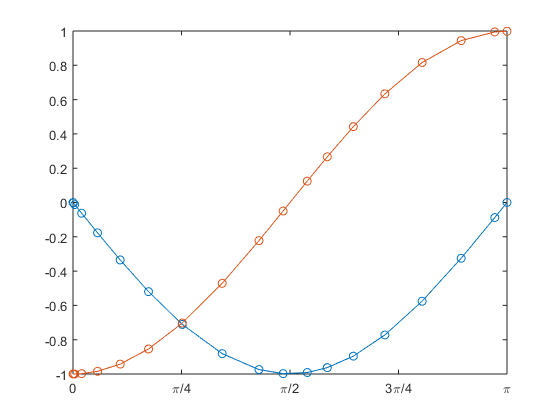# My Favorite ODE

My favorite ordinary differential equation provides a good test of ODE solvers, both numeric and symbolic. It also provides a nice illustration of the underlying existence theory and error analysis.

### Contents

#### Implicit equation

Here is one of my favorite ordinary differential equations. The dot means differentiation with respect to $t$. The equation does not express $\dot{y}$ directly, so it is implicit.

$$\dot{y}^2 + y^2 = 1$$

We don't need a computer to verify that the analytic solution is a combination of $\sin{t}$ and $\cos{t}$. But the constants $y=1$ and $y=-1$ are also solutions.

#### Explicit equation

Our ODE solvers require an explicit equation, but when we solve this equation for $\dot{y}$ we get two solutions, differing in sign.

$$\dot{y} = \pm \sqrt{1-y^2}$$

That $\pm$ sign makes this interesting.

#### First attempt

Let's plunge ahead with the $+$ sign. Specify the equation, an interval for $t$ and an initial condition.

   f = @(t,y) sqrt(1-y^2)
format short
tspan = [0 pi]
y0 = 0

f =
@(t,y)sqrt(1-y^2)
tspan =
0    3.1416
y0 =
0


An attempt to compute a numeric solution with ode45 runs into trouble when $y$ gets slightly larger than $1$ and the square root of a negative number generates an imaginary result. So temporarily use a shorter $t$ interval.

   try
ode45(f,tspan,y0);
catch
ode45(f,[0 1.47],y0)
end
pi_axis([-0.1 1.1])Error using odeplot (line 63)
Error updating the ODEPLOT window.
Solution data may have been corrupted. Argument Y cannot be complex.
Error in ode45 (line 435)


#### Keep in bounds

Change the equation so that it provides a guard against the square root of negative numbers.

   f = @(t,y) sqrt(1-min(y,1)^2)
ode45(f,tspan,y0);
pi_axis([-0.1 1.1])

f =
@(t,y)sqrt(1-min(y,1)^2)This is the correct solution, $\sin{t}$. For a while. But when $y$ reaches $1$ it has to stay there because its derivative is always nonnegative.

#### Switch sign

If we want to continue with $\sin{t}$ beyond $\pi/2$ we have to switch to the minus sign.

   f = @(t,y) (1-2*double(t>pi/2))*real(sqrt(1-y.^2))
ode45(f,tspan,y0)
pi_axis([-0.1 1.1])

f =
@(t,y)(1-2*double(t>pi/2))*real(sqrt(1-y.^2))#### Jacobian

We're solving the autonomous differential equation,

$$\dot{y} = f(y)$$

where

$$f(y) = \sqrt{1-y^2}$$

An important quantity in both the existence theory for ODEs and the error analysis for numeric methods is the Jacobian.

$$J = \frac{\partial{f}}{\partial{y}}$$

In this case $J$ is 1-by-1.

$$J = -\frac{y}{\sqrt{1 - y^2}}$$

When $y$ approaches $\pm 1$ the Jacobian approaches infinity.

Existence and uniqueness theorems for ODEs rely on a bounded Jacobian (or a bounded Lipschitz constant). The derivations of numerical methods rely on a bounded Jacobian. Error control in modern ODE software relies on a bounded Jacobian. All of these are up in the air with this example.

#### Nonuniqueness

We can see the lack of uniqueness happening when we change the initial condition to $y(0) = -1$. The Jacobian is infinite at the start and both the constant $y(t) = -1$ and the trig function $y(t) = -\cos{t}$ are solutions. A small perturbation in the initial condition switches the numerical solution from one to the other.

   f = @(t,y) sqrt(1-max(min(y,1),-1)^2)

y0 = -1
ode45(f,tspan,y0);
pi_axis([-1.2 0])
hold on

format long
y0 = -.99999999
ode45(f,tspan,y0);
pi_axis([-1.1 1.1])
hold off

f =
@(t,y)sqrt(1-max(min(y,1),-1)^2)
y0 =
-1
y0 =
-0.999999990000000#### Symbolic solutions

I was curious to see how the Symbolic Toolbox handles this equation with this initial condition. There is good news and surprising news.

   syms y(t)
ode = diff(y)^2+y^2==1
init = y(0)==-1
ysym = dsolve(ode,init)

ode(t) =
diff(y(t), t)^2 + y(t)^2 == 1
init =
y(0) == -1
ysym =
-1
-cosh(t*1i)


I am pleased to see the two solutions confirmed, but I am surprised to see the second one expressed as a hyperbolic trig function of an imaginary argument.

#### Simplify

Simplification generates a more familiar form.

   simplify(ysym)

ans =
-1
-cos(t)


#### Second order equation

Let's return to the original implicit equation and differentiate the entire equation with respect to $t$.

$$\frac{d}{dt} \left( \dot{y}^2 + y^2 = 1 \right)$$

$$2 \dot{y} \ddot{y} + 2 y \dot{y} = 0$$

We can divide through this equation by $2 \dot{y}$, provided that ${\dot{y}}$ is nonzero. This eliminates the constant solutions and produces an old friend, the harmonic oscillator.

$$\ddot{y} + y = 0$$

#### First order system.

Our ODE solvers need the second order equation to be expressed as a first order system.

$$\dot{y_1} = y_2, \ \ \dot{y_2} = -y_1$$

Now there is no trouble computing $\sin{t}$ and $-\cos{t}$.

   f = @(t,y)[y(2); -y(1)]
y0 = [0; -1]
ode23(f,tspan,y0)
pi_axis([-1 1])

f =
@(t,y)[y(2);-y(1)]
y0 =
0
-1#### pi_axis

I have been using this little function to label the x-axis with multiples of $\pi$ and scale the y-axis.

   type pi_axis

function pi_axis(yaxis)
axis([0 pi yaxis])
set(gca,'xtick',0:pi/4:pi, ...
'xticklabels',{0 '\pi/4' '\pi/2' '3\pi/4' '\pi'})
end


Published with MATLAB® R2016a

|